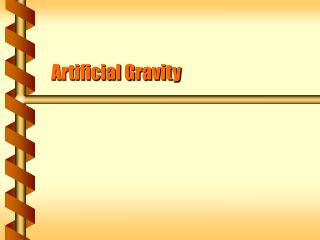Download PresentationArtificial Gravity

Artificial Gravity - PowerPoint PPT Presentation

Artificial Gravity. Central Force. There is no normal or tension force affecting things in orbit. Like falling bodies that accelerate, a satellite accelerates toward the earth. Gravity is a central force. orbital velocity. centripetal acceleration. Satellite. Earth. Force on Mass.I am the owner, or an agent authorized to act on behalf of the owner, of the copyrighted work described.
Download PresentationArtificial Gravity

Download Policy: Content on the Website is provided to you AS IS for your information and personal use and may not be sold / licensed / shared on other websites without getting consent from its author.While downloading, if for some reason you are not able to download a presentation, the publisher may have deleted the file from their server.

- - - - - - - - - - - - - - - - - - - - - - - - - - E N D - - - - - - - - - - - - - - - - - - - - - - - - - -
Presentation Transcript
1. Artificial Gravity

2. Central Force • There is no normal or tension force affecting things in orbit. • Like falling bodies that accelerate, a satellite accelerates toward the earth. • Gravity is a central force. orbital velocity centripetal acceleration Satellite Earth

3. Force on Mass • Newton’s law of acceleration applies to orbits. • Centripetal acceleration times mass is the force of gravity • The situation is the same for the satellite or any object in the satellite. Satellite or astronaut F a Earth

4. The net force of an object in circular orbit matches the centripetal acceleration. This is the same for a freely falling object. Velocity does not change the force or acceleration. Free Fall v Fnet a a = g Fnet = mg v Earth

5. Weightlessness • Objects in free fall exert no normal force. • Fnet = -ma = -mg + FN • If a = g, FN = 0 • The same is true in orbit. • Fnet = mar = Fgrav + FN • If ar = Fgrav/m, FN = 0 • Objects in orbit are weightless.

6. Spin the Station • A spinning station in orbit has a centripetal acceleration of its own. • Acceleration is on the inside pointing inward. • There is a corresponding centripetal force for object on the inside wall. Satellite a F

7. Station Gravity • The centripetal force is like an elevator accelerating upward. • Fnet = mar = FN • The net force must be due to a normal force. • Experience as weight • If rw2 = g then it matches earth’s gravity. Satellite a F

8. Centrifuge • A centrifuge spins to create an artificial gravity. • Liquid separation • Pilot training • Amusement rides

9. Gravitron • The Gravitron is an amusement ride that uses centripetal force. • Rotates at 24 rpm • Diameter is 14 m

10. Centripetal Correction • The spin of the earth creates a centripetal acceleration toward the axis of the earth. • The effect is like accelerating down in an elevator. • Items weigh less at the equator. a = w2r